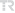## GENERALIZED OSCULATING CURVES OF TYPE (n-3) IN THE n-DIMENSIONAL EUCLIDEAN SPACE

COMMUNICATIONS FACULTY OF SCIENCES UNIVERSITY OF ANKARA-SERIES A1 MATHEMATICS AND STATISTICS, vol.71, no.1, pp.212-225, 2022 (ESCI)• Publication Type: Article / Article
• Volume: 71 Issue: 1
• Publication Date: 2022
• Doi Number: 10.31801/cfsuasmas.845845
• Journal Name:
• Journal Indexes: Emerging Sources Citation Index (ESCI), TR DİZİN (ULAKBİM)
• Page Numbers: pp.212-225
• Keywords: Osculating curve, curvatures, unit speed curve, higher order linear differential equation, RECTIFYING CURVES, TIME-LIKE, PLANE
• Recep Tayyip Erdoğan University Affiliated: Yes

#### Abstract

In this paper, we give a generalization of the osculating curves to the n-dimensional Euclidean space. Based on the definition of an osculating curve in the 3 and 4 dimensional Euclidean spaces, a new type of osculating curve has been defined such that the curve is independent of the (n - 3)th binormal vector in the n-dimensional Euclidean space, which has been called "a generalized osculating curve of type (n-3)". We find the relationship between the curvatures for any unit speed curve to be congruent to this osculating curve in E-n. In particular, we characterize the osculating curves in E-n in terms of their curvature functions. Finally, we show that the ratio of the (n - 1)th and (n - 2)th curvatures of the osculating curve is the solution of an (n - 2)th order linear nonhomogeneous differential equation.